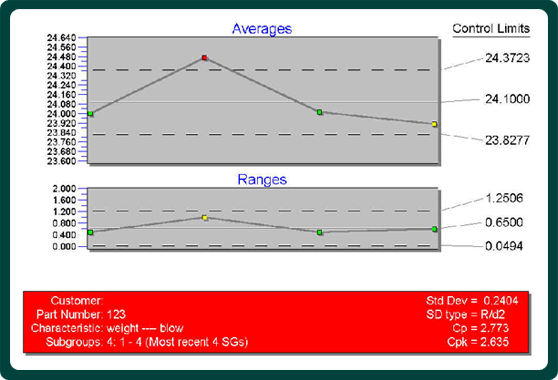Services for the
Plastic Container
Industry

Latest News

ServicesThe experts at Apex Container Tech Inc. are here to assist you in your current business or with starting a new one

# SPC1

A GENERAL PURPOSE PRIMER
The importance of SPC has grown in our industry over the last 15 years to a point where it can no longer be ignored by any blow molding company. There are several approaches to be taken. They depend mostly on what type of business you are in. As a custom blow molder with short runs, it is not feasible to go to the last depths of SPC because by the time we get there, the run has been finished. As an in-house production of a small number of molds on the other side, huge increases in productivity can be gained by fine-tuning the process in great length.
This primer is a start for all engineers that need to get involved in SPC. Depending on your situation you might go much further from here or just do the suggested procedures.
Where to start?
Some of the concepts that we need:

X-bar chart: The average of a parameter such as weight plotted on a x-y axis matrix.

Range chart: The difference between minimum and maximum value of a parameter plotted on a x-y axis matrix.
Variance: A measure of variability calculated by summing up the differences between each value and the mean, then dividing this difference by the number of occurrences.
Standard deviation: Another measure of variability, the square root of variance.
Lower and upper specification limits (LSL and USL): The actual limits that are usually set by the end customer.
Lower and upper control limits (LCL and UCL): The calculated limits of the process calculated by subtracting (adding to for UCL) 3 times the standard deviation from the mean.
cpk: Process capability factor, the smaller of either (USL – Mean of all data)/3 x Standard deviation or (LSL + Mean of all data)/3 x Standard deviation. We are looking for a minimum of 1.33, higher numbers are better.

Below is a sample chart with interpretation underneath.This is a sample of a 4 cavity system. The test parameter is weight.
Looking at the range chart, all cavities are within the control limits and therefore pass.

On the average chart, there is a problem with cavity #2. It is above the control limits and therefore does not pass. Do no be fooled by the relatively high cpk value of 2.635. Because each subgroup consists of only one cavity, the cpk value is usually high. That does not imply that your process is within the control limits. All we are looking for here is to determine if all cavities are statistically the same so that we can take just one cavity as a sample for any subsequent tests. In the case above, reduce the weight on cavity #2 and run the test again

Next month I will show the steps to test if your process is in control.